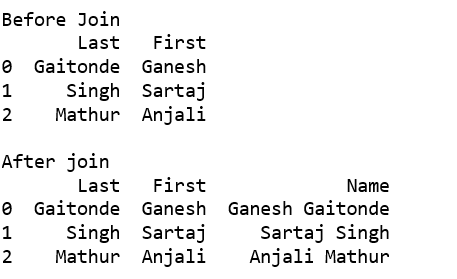# Join two text columns into a single column in Pandas

Let’s see the different methods to join two text columns into a single column.

Method #1: Using cat() function
We can also use different separators during join. e.g. -, _, ” ” etc.

 `# importing pandas ` `import` `pandas as pd ` ` `  `df ``=` `pd.DataFrame({``'Last'``: [``'Gaitonde'``, ``'Singh'``, ``'Mathur'``], ` `                   ``'First'``: [``'Ganesh'``, ``'Sartaj'``, ``'Anjali'``]}) ` ` `  `print``(``'Before Join'``) ` `print``(df, ``'\n'``) ` ` `  `print``(``'After join'``) ` `df[``'Name'``] ``=` `df[``'First'``].``str``.cat(df[``'Last'``], sep ``=``" "``) ` `print``(df) `

Output :Method #2: Using lambda function

This method generalizes to an arbitrary number of string columns by replacing df[[‘First’, ‘Last’]] with any column slice of your dataframe, e.g. df.iloc[:, 0:2].apply(lambda x: ‘ ‘.join(x), axis=1).

 `# importing pandas ` `import` `pandas as pd ` ` `  `df ``=` `pd.DataFrame({``'Last'``: [``'Gaitonde'``, ``'Singh'``, ``'Mathur'``], ` `                   ``'First'``: [``'Ganesh'``, ``'Sartaj'``, ``'Anjali'``]}) ` ` `  `print``(``'Before Join'``) ` `print``(df, ``'\n'``) ` ` `  `print``(``'After join'``) ` `df[``'Name'``] ``=` `df[[``'First'``, ``'Last'``]].``apply``(``lambda` `x: ``' '``.join(x), axis ``=` `1``) ` `print``(df) `

Output :Method #3: Using + operator

We need to convert data frame elements into string before join. We can also use different separators during join, e.g. -, _, ‘ ‘ etc.

 `# importing pandas ` `import` `pandas as pd ` ` `  `df ``=` `pd.DataFrame({``'Last'``: [``'Gaitonde'``, ``'Singh'``, ``'Mathur'``], ` `                   ``'First'``: [``'Ganesh'``, ``'Sartaj'``, ``'Anjali'``]}) ` ` `  `print``(``'Before Join'``) ` `print``(df, ``'\n'``) ` ` `  `print``(``'After join'``) ` `df[``'Name'``]``=` `df[``"First"``].astype(``str``) ``+``" "``+` `df[``"Last"``] ` `print``(df) `

Output :My Personal Notes arrow_drop_upCheck out this Author's contributed articles.

If you like GeeksforGeeks and would like to contribute, you can also write an article using contribute.geeksforgeeks.org or mail your article to contribute@geeksforgeeks.org. See your article appearing on the GeeksforGeeks main page and help other Geeks.

Please Improve this article if you find anything incorrect by clicking on the "Improve Article" button below.

Article Tags :

Be the First to upvote.

Please write to us at contribute@geeksforgeeks.org to report any issue with the above content.